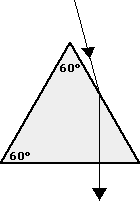Refraction and the Ray Model of Light - Lesson 2 - The Mathematics of Refraction

# Determination of n Values

In a previous part of Lesson 2, we learned about a mathematical equation relating the two angles (angles of incidence and refraction) and the indices of refraction of the two materials on each side of the boundary. The equation is known as the Snell's Law equation and is expressed as follows.

 ni • sine(Θi) = nr • sin(Θr) where Θi ("theta i") = angle of incidence Θr ("theta r") = angle of refraction ni = index of refraction of the incident medium nr = index of refraction of the refractive medium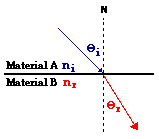As with any equation in physics, the Snell's Law equation is valued for its predictive ability. If any three of the four variables in the equation are known, the fourth variable can be predicted if appropriate problem-solving skills are employed. The task of using the equation to solve several varieties of problems was thoroughly discussed in a previous part of this Lesson 2. In a similar manner, the equation can be used to determine the index of refraction of a material if the path of light through the material is known. In this part of Lesson 2, we will investigate the details of this task.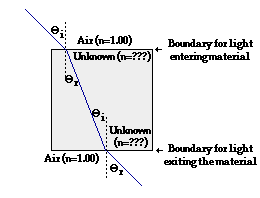A common lab performed in a Physics class involves the determination of the index of refraction of an unknown material. Typically, a series of transparent objects (glass and Lucite squares, rectangles and triangles) made of an unknown material are distributed and the task is assigned to determine the index of refraction of each unknown material. Of course, the task of determining the index of refraction value of an unknown material would be easy if the other three quantities in the Snell's Law equation are known. Thus, a line of sight method or a laser beam is used to determine the path of the light into the material, through the material and out of the material to the students' eyes. The diagram depicts a typical path of one such ray through a square block. Observe that the light encounters two boundaries - the boundary upon entering the glass and the boundary upon exiting the glass. The ray of light refracts at each boundary. By measuring the angles of incidence and refraction and using the index of refraction of air, the index of refraction of the unknown material can be found. Calculations can be performed at each boundary and the results can be averaged. The data table below represents sample data for the Index of Refraction Lab; the listed values correspond to the diagram at the right.

Sample Data

 Θi Θr sine Θi sine Θr Calculated n value Light Ray Entering Material 45 deg. 24 deg. 0.7071 0.4067 1.74 Light Ray Exiting Material 24 deg. 45 deg. 0.4067 0.7071 1.74 Ave. n Value ---> 1.74

The accompanying work for this lab is as follows:

First Boundary: Light Ray Entering Material
 Diagram: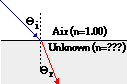Known: ni = 1.00 (from table) Θi = 45 degrees Θr = 24 degrees Find: nr = ???

ni • sine(Θi) = nr • sin(Θr)

1.00 * sine(45 deg.) = nr * sine(24 deg.)

0.7071 = 0.4067 * nr

(0.7071)/(0.4067) = nr

1.74 = nr

Second Boundary: Light Ray Exiting Material

 Diagram: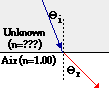Known: nr = 1.00 (from table) Θr = 45 degrees Θi = 24 degrees Find: ni = ???

ni • sine(Θi) = nr • sin(Θr)

ni * sine(24 deg.) = 1.000 * sine(45 deg.)

ni * 0.4067 = 0.7071

ni = (0.7071)/(0.4067)

ni = 1.74

Observe in the data table above the two calculated n values are the same. This should not be a surprise since the unknown material only has one index of refraction value. If different values were obtained, it would be the result of some form of measurement error in the retrieval of data.

This method of determining the index of refraction of an unknown material is one more application of the Snell's Law equation. Test your ability to use the equation in this manner by answering the following questions.

### We Would Like to Suggest ...Why just read about it and when you could be interacting with it? Interact - that's exactly what you do when you use one of The Physics Classroom's Interactives. We would like to suggest that you combine the reading of this page with the use of our Refraction Interactive. You can find it in the Physics Interactives section of our website. The Refraction Interactive provides the learner an interactive enivronment for exploring the refraction and reflection of light at a boundary between two materials.

1. Cal Culator is performing an experiment to determine the index of refraction of an unknown material (in the shape of a 45-45-90 triangle). Cal determines that the light follows the path as shown on the diagram below. Use this path, a protractor, a calculator and Snell's Law to determine the index of refraction of the unknown material.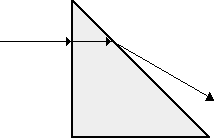2. The path of a light ray through an unknown material is shown in the diagram below. Make some measurements and determine the index of refraction of the material.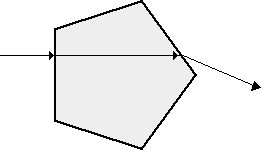3. Light traveling through air (n = 1.00) is incident upon a triangular block made of an unknown material. The path of the light through the material is shown in the diagram below. Using a protractor and a calculator, determine the index of refraction of the unknown material.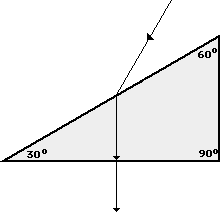4. Light traveling through air (n = 1.00) is incident upon a 60-60-60 triangular block (the triangle is equilateral; the sides make 60-degree angles with each other) made of an unknown material. The path of the light through the material is shown in the diagram below. Using a protractor and a calculator, determine the index of refraction of the unknown material.